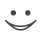# Get these flashcards, study & pass exams. For free! Even on iPhone/Android!

## Function Shifts (10 Cards)

y = |x| + 2
A V-shaped graph shifted up 2 units
f(x)=(x+3)^2
Parabola shifted 3 units left.
f(x)=√x-6
The square-root function shifted 6 units down
f(x) = |x + 2| - 4
A V-shaped graph shifted left 2 units and down 4 units
y = f(x) + k
The graph of f(x) shifted

up k units if k is positive

down k units if k is negative
y = f(x - h)
The graph of f(x) shifted

left h units if h is negative  (might look like y = f(x + h)...)

right h units if h is positive
f(x)=√(x+2)
The graph of the square-root function shifted left 2 units
y = |x - 6| + 1
A V-shaped graph shifted 6 units right and 1 unit up.
f(x)=(x-3)^2 - 5
A parabola shifted 3 units right and 5 units down
f(x)=√(x+4) + 2
The square-root function shifted 4 units left and 2 units up.Flashcard set info:
Author: chris
Main topic: Mathematics
Topic: Functions
Published: 02.07.2010

Card tags:
All cards (10)
no tagsReport abuse